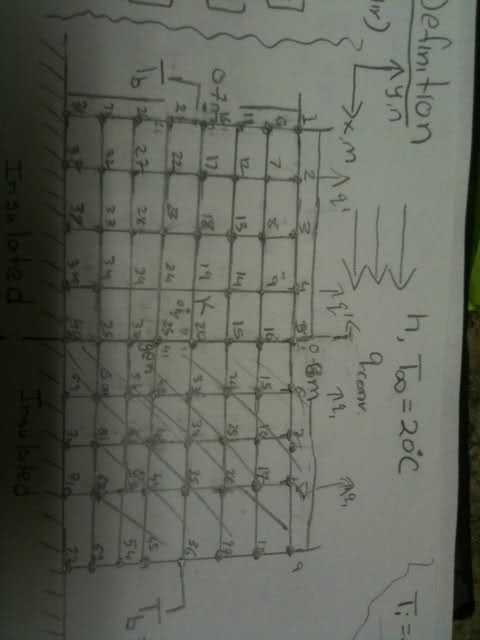# 2D Heat Conduction - MATLAB help

Hi all, I am working on the problem below, and I wrote the code, but it's not working. Can anyone help me out? And even any ideas on how to improve the code to make it more succinct?

http://i25.tinypic.com/2v0zi8m.jpgBasically, it is a 2D conduction problem with convection heat transfer on the top, insulated at the bottom edge, and temperature held constant at the left and right edge. The dimensions of the plate are 0.8x0.7 with dx=dy=dx=0.1. I used the symmetry, and the just worked on the left side of the symmetry line (nodes 1-40), wrote nodal equations (finite difference eqs) for each node, and then created a 40x40 matrix in matlab to solve system of unknown temperatures. However, my answer is not even remotely close. It doesn't matter what values I choose for k, h, Tb, q_dot, or any other constants. Here's my code. It's very long. I hope there's an easier way to implement this. IF there is, can someone help me or give me any ideas?

clc
clear

%Material Properties & Constants
k = 205;
h = 10;
qdot = 1000;
Tinf = 293.15;
Tb = 423.15;
dx = 0.1;
c = (-qdot*dx^2)/k;
d = 2*((h*dx)/k)*Tinf;
%Matrices
n = 5*8;
a = zeros(n,n);
b = zeros(n);

%node(1)
a(1,1)=-2*((h*dx)/k+1);
a(1,2)=1;
a(1,6)=1;
b(1)=c-d;

%node(2)
a(2,2)=-2*((h*dx)/k+2);
a(2,1)=1;
a(2,3)=1;
a(2,7)=2;
b(2)=c-d;

%node(3)
a(3,3)=-2*((h*dx)/k+2);
a(3,2)=1;
a(3,4)=1;
a(3,8)=2;
b(3)=c-d;

%node(4)
a(4,4)=-2*((h*dx)/k+2);
a(4,3)=1;
a(4,5)=1;
a(4,9)=2;
b(4)=c-d;

%node(5)
a(5,5)=-2*((h*dx)/k+2);
a(5,4)=2;
a(5,10)=2;
b(5)=c-d;

%node(6)
a(6,6)=Tb;
b(6)=0;

%node(7)
a(7,7)=-4;
a(7,2)=1;
a(7,6)=1;
a(7,8)=1;
a(7,12)=1;
b(7)=c;

%node(8)
a(8,8)=-4;
a(8,3)=1;
a(8,7)=1;
a(8,9)=1;
a(8,13)=1;
b(8)=c;

%node(9)
a(9,9)=-4;
a(9,4)=1;
a(9,8)=1;
a(9,10)=1;
a(9,14)=1;
b(9)=c;

%node(10)
a(10,10)=-4;
a(10,5)=1;
a(10,9)=2;
a(10,15)=1;
b(10)=c;

%node(11)
a(11,11)=Tb;
b(11)=0;

%node(12)
a(12,12)=-4;
a(12,7)=1;
a(12,11)=1;
a(12,13)=1;
a(12,17)=1;
b(12)=c;

%node(13)
a(13,13)=-4;
a(13,8)=1;
a(13,12)=1;
a(13,14)=1;
a(13,18)=1;
b(13)=c;

%node(14)
a(14,14)=-4;
a(14,8)=1;
a(14,13)=1;
a(14,15)=1;
a(14,19)=1;
b(14)=c;

%node(15)
a(15,15)=-4;
a(15,10)=1;
a(15,14)=2;
a(15,20)=1;
b(15)=c;

%node(16)
a(16,16)=Tb;
b(16)=0;

%node(17)
a(17,17)=-4;
a(17,12)=1;
a(17,16)=1;
a(17,18)=1;
a(17,22)=1;
b(17)=c;

%node(18)
a(18,18)=-4;
a(18,13)=1;
a(18,17)=1;
a(18,19)=1;
a(18,23)=1;
b(18)=c;

%node(19)
a(19,19)=-4;
a(19,14)=1;
a(19,18)=1;
a(19,20)=1;
a(19,24)=1;
b(19)=c;

%node(20)
a(20,20)=-4;
a(20,15)=1;
a(20,19)=2;
a(20,25)=1;
b(20)=c;

%node(21)
a(21,21)=Tb;
b(21)=0;

%node(22)
a(22,22)=-4;
a(22,17)=1;
a(22,21)=1;
a(22,23)=1;
a(22,27)=1;
b(22)=c;

%node(23)
a(23,23)=-4;
a(23,18)=1;
a(23,22)=1;
a(23,24)=1;
a(23,28)=1;
b(23)=c;

%node(24)
a(24,24)=-4;
a(24,19)=1;
a(24,23)=1;
a(24,25)=1;
a(24,29)=1;
b(24)=c;

%node(25)
a(25,25)=-4;
a(25,20)=1;
a(25,24)=2;
a(25,30)=1;
b(25)=c;

%node(26)
a(26,26)=Tb;
b(26)=0;

%node(27)
a(27,27)=-4;
a(27,22)=1;
a(27,26)=1;
a(27,28)=1;
a(27,32)=1;
b(27)=c;

%node(28)
a(28,28)=-4;
a(28,23)=1;
a(28,27)=1;
a(28,29)=1;
a(28,33)=1;
b(28)=c;

%node(29)
a(29,29)=-4;
a(29,24)=1;
a(29,28)=1;
a(29,30)=1;
a(29,34)=1;
b(29)=c;

%node(30)
a(30,30)=-4;
a(30,25)=1;
a(30,29)=2;
a(30,35)=1;
b(30)=c;

%node(31)
a(31,31)=Tb;
b(31)=0;

%node(32)
a(32,32)=-4;
a(32,27)=1;
a(32,31)=1;
a(32,33)=1;
a(32,37)=1;
b(32)=c;

%node(33)
a(33,33)=-4;
a(33,28)=1;
a(33,32)=1;
a(33,34)=1;
a(33,38)=1;
b(33)=c;

%node(34)
a(34,34)=-4;
a(34,29)=1;
a(34,33)=1;
a(34,35)=1;
a(34,39)=1;
b(34)=c;

%node(35)
a(35,35)=-4;
a(35,30)=1;
a(35,34)=2;
a(35,40)=1;
b(35)=c;

%node(36)
a(36,36)=Tb;
b(36)=0;

%node(37)
a(37,37)=-4;
a(37,32)=2;
a(37,36)=1;
a(37,38)=1;
b(37)=c;

%node(38)
a(38,38)=-4;
a(38,33)=2;
a(38,37)=1;
a(38,39)=1;
b(38)=c;

%node(39)
a(39,39)=-4;
a(39,34)=2;
a(39,38)=1;
a(39,40)=1;
b(39)=c;

%node(40)
a(40,40)=-4;
a(40,35)=2;
a(40,39)=2;
b(40)=c;

T=a\b;

fprintf(' Forcing Function Vector \n ')
b(:,1)
fprintf(' NODE TEMPERATURE (K) \n ')
TSolution=T(:,1);

contourf(T)

for i=1:length(TSolution)
fprintf(' %4.0f \t \t %6.1f \n',i,TSolution(i))
end

## Answers and Replies

One of the problems that you may be having is:

%node(16)
a(16,16)=Tb;
b(16)=0;

I think that this should be:

%node(16)
a(16,16)=1;
b(16)=Tb;

so the equation that you would get in this case would be:

1 x T16 = Tb
so T16 = Tb/1 = Tb

In your case:

Tb x T16 = 0
so T16 = 0/Tb = 0

Question:Why u put C-D at node 1 until node 5?